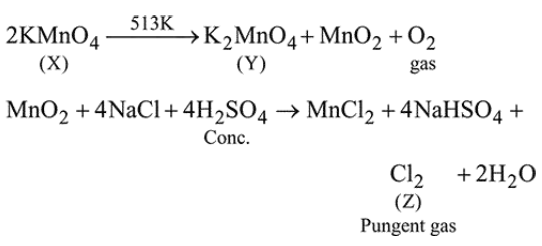# Thermal decomposition of a Mn compound (X) atQuestion:

Thermal decomposition of a Mn compound (X) at $513 \mathrm{~K}$ results in compound $\mathrm{Y}, \mathrm{MnO}_{2}$ and a gaseous product. $\mathrm{MnO}_{2}$ reacts with $\mathrm{NaCl}$ and concentrated $\mathrm{H}_{2} \mathrm{SO}_{4}$ to give a pungent gas Z. X, Y, and Z, respectively, are :

1. $\mathrm{K}_{3} \mathrm{MnO}_{4}, \mathrm{~K}_{2} \mathrm{MnO}_{4}$ and $\mathrm{Cl}_{2}$

2. $\mathrm{K}_{2} \mathrm{MnO}_{4}, \mathrm{KMnO}_{4}$ and $\mathrm{SO}_{2}$

3. $\mathrm{KMnO}_{4}, \mathrm{~K}_{2} \mathrm{MnO}_{4}$ and $\mathrm{Cl}_{2}$

4. $\mathrm{K}_{2} \mathrm{MnO}_{4}, \mathrm{KMnO}_{4}$ and $\mathrm{Cl}_{2}$

Correct Option: 1

Solution: